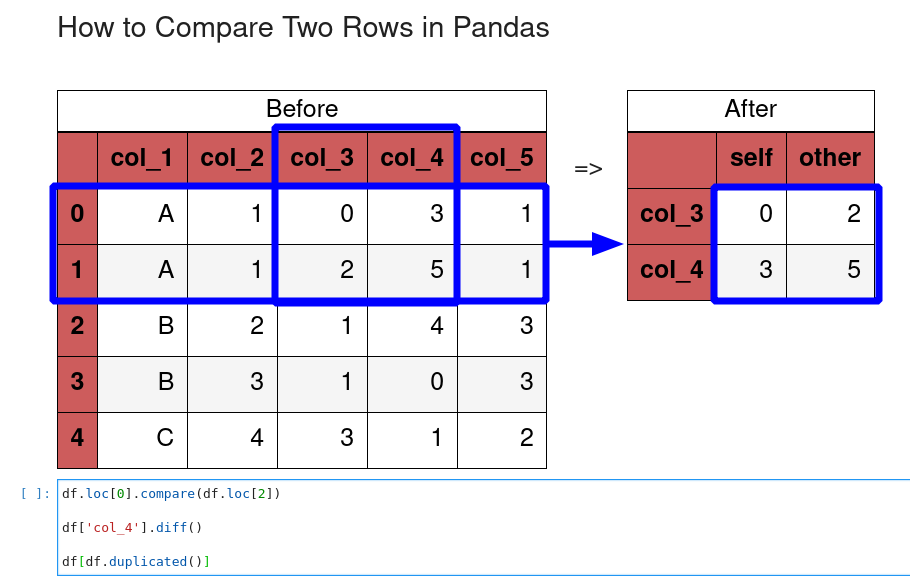In this short guide, we'll see how to compare rows in Pandas DataFrame. We will also cover how to find the difference between two rows in Pandas.

To compare columns or DataFrames in Pandas please refer to:

## 2. Setup

In the post, we'll use the following DataFrame, which consists of several rows and columns:

``````import pandas as pd
data = [('A',1, 0, 3, 1),
('A',1, 2, 5, 1),
('B',2, 1, 4, 3),
('B',3, 1, 0, 3),
('C',4, 3, 1, 2)]
cols = ('col_1', 'col_2', 'col_3', 'col_4', 'col_5' )
df = pd.DataFrame(data,
columns = cols)
``````

Data is:

col_1 col_2 col_3 col_4 col_5
0 A 1 0 3 1
1 A 1 2 5 1
2 B 2 1 4 3
3 B 3 1 0 3
4 C 4 3 1 2

## Step 1: Compare two rows

Pandas offers the method `compare()` which can be used in order of two rows in Pandas.

Let's check how we can use it to compare specific rows in DataFrame. We are going to compare row with index - 0 to row - 2:

``````df.loc.compare(df.loc)
``````

The result is all values which has difference:

self other
col_1 A B
col_2 1 2
col_3 0 1
col_4 3 4
col_5 1 3

Comparing the first two rows will give us only the differences:

self other
col_3 0 2
col_4 3 5## Step 2: Compare two rows with highlighting

To compare 2 rows while showing highlighted differences can be achieved with a custom function.

First we will select the rows for comparison and transpose the resulting DataFrame by `df.loc[:1].T`. Then we will apply the function for comparison:

``````def highlight(d):
df = pd.DataFrame(columns=d.columns, index=d.index)

col1 = d.columns
col2 = d.columns
df[[col1, col2]] = 'background: None'

df.loc[d[col1].ne(d[col2]), [col1, col2]] = 'background: yellow'

return df

df.loc[:1].T.style.apply(highlight, axis=None)
``````

the result is:

0 1
col_1 A A
col_2 1 1
col_3 0 2
col_4 3 5
col_5 1 1

## Step 3: Calculate the difference between rows

To calculate the difference between rows - row by row we can use the function - `diff()`. To calculated difference for `col_4`:

``````df['col_4'].diff()
``````

the result would be difference calculated for each two rows:

``````0    NaN
1    2.0
2   -1.0
3   -4.0
4    1.0
Name: col_4, dtype: float64
``````

Method `diff()` has two useful parameters:

• `periods` - shift of periods for calculating difference
• `axis` - rows or columns

If there are string values in some rows you may face the following error:

TypeError: unsupported operand type(s) for -: 'str' and 'str'

## Step 4: Compare rows from different DataFrames

In order to compare rows from different DataFrames we can select them by index:

``````df2 = df.copy()
df.loc.compare(df2.loc)
``````

Another option is to match rows on another column - let say unique column - `url`. We can match the records by:

``````url_same = df1[df1.url.isin(df2.url)].url
``````

Then we can select one by:

``````url = url_same
``````

Finally we can concatenated into single DataFrame as:

``````pd.concat([df1[df1.url == url], df2[df2.url == url]]).T
``````

Finally we can transpose the result for readability. The full code:

``````url_same = df1[df1.url.isin(df2.url)].url
url = url_same

df_c = pd.concat([df1[df1.url == url], df2[df2.url == url]]).T
``````

## Step 5: Finding the duplicate rows

We can find the duplicated rows in the whole DataFrame by command like:

``````df[['col_1', 'col_2']].duplicated()
``````

Method `duplicated()` returns boolean array for duplicate rows:

``````0    False
1     True
2    False
3    False
4    False
dtype: bool
``````

or for the whole DataFrame. In this case we will get also the data:

``````df[df.duplicated()]
``````

Empty DataFrame since there no duplicated rows on every column

## Step 6: Finding the unique rows

To find all the unique rows we can use:

``````df.drop_duplicates()
``````

or for subset of columns:

``````df[['col_1', 'col_2']].drop_duplicates()
``````

result:

col_1 col_2
0 A 1
2 B 2
3 B 3
4 C 4

## Conclusion

In this article we show how to compare rows, compare rows with highlighting and extract the difference of two rows. We covered comparison of rows from different DataFrames and calculating the difference between rows for the whole DataFrame.